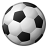# Adding impedance to edge of road net work data set from another shapefile 'z' value

719
4
08-17-2010 10:33 AMby
New Contributor
I am looking for an optimum path where road/edge impedance is a variable and that is available in shape file formate (x(longitude),y(latitude),z(value)). I want to add z value to network data set as impedance. Kindly help me.

Thanks....
Tags (2)
4 RepliesbyEsri Contributor
In order to add the appropriate z value as impedance, you will have to first transfer the Z value from the shape field to a regular field (most likely of type double) in your streets shapefile. Then in your network dataset, you can create a network cost attribute based on this field.

There are multiple ways to transfer the Z value from shape field to an attribute. Before suggesting them, I would want to know

1. What ArcGIS version you are using?

2. Do you also have a license for 3D Analyst Extension?

3. Does your lines have the same Z value for all vertices that make up a line? If not, then do you want to store mean Z, or max Z or min Z for each line?

Deeleshby
New Contributor
In order to add the appropriate z value as impedance, you will have to first transfer the Z value from the shape field to a regular field (most likely of type double) in your streets shapefile. Then in your network dataset, you can create a network cost attribute based on this field.

There are multiple ways to transfer the Z value from shape field to an attribute. Before suggesting them, I would want to know

1. What ArcGIS version you are using?

2. Do you also have a license for 3D Analyst Extension?

3. Does your lines have the same Z value for all vertices that make up a line? If not, then do you want to store mean Z, or max Z or min Z for each line?

Deelesh

My ArcGIS version is 9.1 and have license for 3D Analyst Extension.
Z value is not constant and it is a function of latitude and longitude.
I want to store average z which are near to the road segment.

RomalbyEsri Contributor
Here are the steps to add the average Z value for each street as an attribute. You will have to unzip the MeanZ.zip file to get the MeanZ.cal field calculator expression file.

1. Add a new field called "AverageZ" of type Double to your line shapefile

2. Right-Click the field and select "Calculate Values"

3. In the Field Calculator dialog, Click the Load button and browse to the  MeanZ.cal expression file.

4. This will load the VBA script that does the calculations to determine average Z. Click OK to run the script.

The script is getting the Z values from each vertex that make up a line and then calculating the average z value.

The script's VBA code

```Dim meanZ as Double
Dim totalZ as Double
Dim pointCount as Double
Dim pLine as IPointCollection
Dim pPoint as IPoint
Dim i as Integer
Set pLine = [Shape]
pointCount = pLine.PointCount
For i = 0 to pointCount -1
set pPoint = pLine.Point(i)
totalZ = totalZ + pPoint.Z
Next
meanZ = totalZ / pointCount
```

For others that might come across this thread, Field Calculator at ArcGIS 10 no longer supports the ability to run VBA scripts. So this same workflow can be achieved by writing a python script or by using the Add Z information geoprocessing tool available with 3D Analyst Extension.

Deeleshby
New Contributor
Here are the steps to add the average Z value for each street as an attribute. You will have to unzip the MeanZ.zip file to get the MeanZ.cal field calculator expression file.

1. Add a new field called "AverageZ" of type Double to your line shapefile

2. Right-Click the field and select "Calculate Values"

3. In the Field Calculator dialog, Click the Load button and browse to the  MeanZ.cal expression file.

4. This will load the VBA script that does the calculations to determine average Z. Click OK to run the script.

The script is getting the Z values from each vertex that make up a line and then calculating the average z value.

The script's VBA code

```Dim meanZ as Double
Dim totalZ as Double
Dim pointCount as Double
Dim pLine as IPointCollection
Dim pPoint as IPoint
Dim i as Integer
Set pLine = [Shape]
pointCount = pLine.PointCount
For i = 0 to pointCount -1
set pPoint = pLine.Point(i)
totalZ = totalZ + pPoint.Z
Next
meanZ = totalZ / pointCount
```

For others that might come across this thread, Field Calculator at ArcGIS 10 no longer supports the ability to run VBA scripts. So this same workflow can be achieved by writing a python script or by using the Add Z information geoprocessing tool available with 3D Analyst Extension.

Deelesh

Thanks Deelesh
The code gives an error message "User Interrupt" .
The Z values are in another shapefile/table .I want to calculate average Z value from the nearest Z values lying on either side of street and assign the average Z value to the street (another shape file)as attribute.